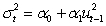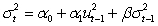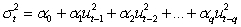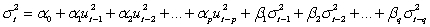# [Solved] What Are the Names of the Following Models

Question 5
Multiple Choice

## What are the names of the following models? (I)(II)(III)(IV)A) GARCH (1), ARCH (1,1), GARCH(q) and ARCH(p,q), respectively
B) ARCH(1), GARCH(1,1), ARCH(q) and GARCH(p,q), respectively
C) ARCH(1), EGARCH(1,1), ARCH(q) and EGARCH(p,q), respectively
D) EGARCH (1), ARCH(1,1), EGARCH (q) and ARCH(p,q), respectively

10+ million students use Quizplus to study and prepare for their homework, quizzes and exams through 20m+ questions in 300k quizzes.

Explore our library and get Economics Homework Help with various study sets and a huge amount of quizzes and questions

3.5K

Study sets

66.5K

Quizzes

5.9M

Questions

Upload material to get free accessInvite a friend and get free accessSubscribe and get an instant access